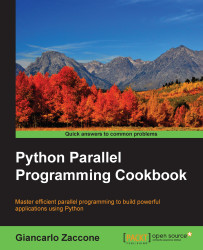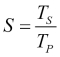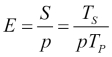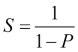•#### Python Parallel Programming Cookbook#### Overview of this book

This book will teach you parallel programming techniques using examples in Python and will help you explore the many ways in which you can write code that allows more than one process to happen at once. Starting with introducing you to the world of parallel computing, it moves on to cover the fundamentals in Python. This is followed by exploring the thread-based parallelism model using the Python threading module by synchronizing threads and using locks, mutex, semaphores queues, GIL, and the thread pool. Next you will be taught about process-based parallelism where you will synchronize processes using message passing along with learning about the performance of MPI Python Modules. You will then go on to learn the asynchronous parallel programming model using the Python asyncio module along with handling exceptions. Moving on, you will discover distributed computing with Python, and learn how to install a broker, use Celery Python Module, and create a worker. You will understand anche Pycsp, the Scoop framework, and disk modules in Python. Further on, you will learnGPU programming withPython using the PyCUDA module along with evaluating performance limitations.
Python Parallel Programming CookbookCreditswww.PacktPub.comPrefaceFree Chapter
Getting Started with Parallel Computing and PythonProcess-based ParallelismAsynchronous ProgrammingDistributed PythonGPU Programming with PythonIndex## How to evaluate the performance of a parallel program

The development of parallel programming created the need of performance metrics and a software tool to evaluate the performance of a parallel algorithm in order to decide whether its use is convenient or not. Indeed, the focus of parallel computing is to solve large problems in a relatively short time. The factors that contribute to the achievement of this objective are, for example, the type of hardware used, the degree of parallelism of the problem, and which parallel programming model is adopted. To facilitate this, analysis of basic concepts was introduced, which compares the parallel algorithm obtained from the original sequence. The performance is achieved by analyzing and quantifying the number of threads and/or the number of processes used.

To analyze this, a few performance indexes are introduced: speedup, efficiency, and scaling.

The limitations of a parallel computation are introduced by the Ahmdal's law to evaluate the degree of the efficiency of parallelization of a sequential algorithm we have the Gustafson's law.

### Speedup

Speedup is the measure that displays the benefit of solving a problem in parallel. It is defined as the ratio of the time taken to solve a problem on a single processing element, TS, to the time required to solve the same problem on p identical processing elements, Tp.

We denote speedup by. We have a linear speedup, where if S=p, it means that the speed of execution increases with the number of processors. Of course, this is an ideal case. While the speedup is absolute when Ts is the execution time of the best sequential algorithm, the speedup is relative when Ts is the execution time of the parallel algorithm for a single processor.

Let's recap these conditions:

• S = p is linear or ideal speedup

• S < p is real speedup

• S > p is superlinear speedup

### Efficiency

In an ideal world, a parallel system with p processing elements can give us a speedup equal to p. However, this is very rarely achieved. Usually, some time is wasted in either idling or communicating. Efficiency is a performance metric estimating how well-utilized the processors are in solving a task, compared to how much effort is wasted in communication and synchronization.

We denote it by E and can define it as. The algorithms with linear speedup have the value of E = 1; in other cases, the value of E is less than `1`. The three cases are identified as follows:

• When E = 1, it is a linear case

• When E < 1, it is a real case

• When E<< 1, it is a problem that is parallelizable with low efficiency

### Scaling

Scaling is defined as the ability to be efficient on a parallel machine. It identifies the computing power (speed of execution) in proportion with the number of processors. By increasing the size of the problem and at the same time the number of processors, there will be no loss in terms of performance. The scalable system, depending on the increments of the different factors, may maintain the same efficiency or improve it.

### Amdahl's law

Amdahl's law is a widely used law used to design processors and parallel algorithms. It states that the maximum speedup that can be achieved is limited by the serial component of the program:, where 1 – P denotes the serial component (not parallelized) of a program. This means that for, as an example, a program in which 90 percent of the code can be made parallel, but 10 percent must remain serial, the maximum achievable speedup is `9` even for an infinite number of processors.

### Gustafson's law

Gustafson's law is based on the following considerations:

• While increasing the dimension of a problem, its sequential parts remain constant

• While increasing the number of processors, the work required on each of them still remains the same

This states that S(P) = P – α ( P – 1), where P is the number of processors, S is the speedup, and α is the non-parallelizable fraction of any parallel process. This is in contrast to Amdahl's law, which takes the single-process execution time to be the fixed quantity and compares it to a shrinking per process parallel execution time. Thus, Amdahl's law is based on the assumption of a fixed problem size; it assumes that the overall workload of a program does not change with respect to the machine size (that is, the number of processors). Gustafson's law addresses the deficiency of Amdahl's law, which does not take into account the total number of computing resources involved in solving a task. It suggests that the best way to set the time allowed for the solution of a parallel problem is to consider all the computing resources and on the basis of this information, it fixes the problem.